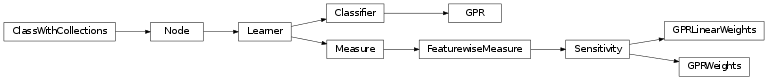# mvpa2.clfs.gpr¶

Gaussian Process Regression (GPR).Functions

Classes

 `GPR`([kernel]) Gaussian Process Regression (GPR). `GPRLinearWeights`(clf[, force_train]) `SensitivityAnalyzer` that reports the weights GPR trained `GPRWeights`(clf[, force_train]) `SensitivityAnalyzer` that reports the weights GPR trained

Exceptions

 `GPR`([kernel]) Gaussian Process Regression (GPR). `GPRLinearWeights`(clf[, force_train]) `SensitivityAnalyzer` that reports the weights GPR trained `GPRWeights`(clf[, force_train]) `SensitivityAnalyzer` that reports the weights GPR trained